## Please enable JavaScript to view this site.

 Navigation: MTune Settings > CAN Bus CAN Inputs

Vehicle CAN data can be used in MaxxECU for trigger certain functions, or log data if CAN ID are known.

A CAN analyzer must be used to "sniff" vehicle CAN, if the CAN ID/data are not known, example of a good and simple CAN analyzer: www.cananalyser.co.uk/

CAN input value

Enable

disabled

Enable, CAN message input - receive CAN messages, used as any analog class or bit mask to be used as a Digital inputs signal.

Enable, obd2 input - Request ODB2 PID data directly into this CAN input system, received into any analog class or bit mask to be used as a Digital inputs signal.

CAN Bus

Specifies which CAN bus to use (more than one CAN bus is only available in MaxxECU PRO).

CAN message ID

Message ID (decimal value) to be used.

ODB2 PID

The OBD2 PID to request, see available PIDs, wikipedia OBD-II_PIDs.

ODB2 ECU

The response ID the system is listening to.

ODB2 request rate

Specifies the requested update rate of the ODB2 PID requested value.OBD2 PID examples

timeout

timeout

Whether to use a timeout on this particular message. If no message has been received within the timeout time, the value is zeroed out.

timeout time

Specifies the time to wait for a message. Specified in seconds.

Data

ByteOffset

Byte offset to use. Example: second byte, offset = 1.

Endian

Big-endian and little-endian are terms that describe the order in which a sequence of bytes are stored in computer memory.

Big-endian is an order in which the "big end" (most significant value in the sequence) is stored first (at the lowest storage address).

Little-endian is an order in which the "little end" (least significant value in the sequence) is stored first.

Type

Signed variables, such as signed integers will allow you to represent numbers both in the positive and negative ranges.

Unsigned variables, such as unsigned integers, will only allow you to represent positive numbers.

Mask operation applied before value conversion. Logical AND operation.

0xFFF to only use the first 12-bits of a 16-bit field for example.

Value conversion (analog)

### Value conversion mathematical formula

Value = 0.1*(Indata+Offset)*Multiplier/Divider

offset

Offset to use in the conversion formula.

Multiplier

Multiplier to use in the conversion formula.

Divider

Divider to use in the conversion formula.

Note: see below current value top check your final value to be used in MaxxECU.

resolution

Specifies the sensor resolution on the selected input.

1 (-32768 to +32767) - Values between -32768 and + 32767 can be used on the table (no decimals).

0.1 (-3276.8 to +3276.7) - Values between -3276.8 and + 3276.7 can be used on the table (one decimal).

0.01 (-327.68 to +327.67) - Values between -327.68 and + 327.67 can be used on the table (two decimals).

0.001 (-32.768 to +32.767) - Values between -32.768 and + 32.767 can be used on the table (three decimals).

Value usage (analog)

name

Name of the converted value. Visible in MTune.

unit

Unit for the converted value. Visible in MTune.

destination

Value will be visible in RealTime Data --> User CAN inputs.

Can be used in MaxxECU as any other RealTime value for conditions or warning. For example it can be used to read GPS SPEED from CAN and use as a wheelspeed input.

Current value

The final value after all conversion, this value is to be used in MaxxECU.

Value usage (digital)

Defines which bits in the byte you want to keep, and which bits you want to clear. MaxxECU here uses bitwise AND in order to extract a subset of the bits in the value.

To set the input, (value & bit mask) cant be "0" (>=1) to activate the MaxxECU digital input function below.

(Bit is either "0" or "1", and 8 bits grouped together makes 1 byte).

We can use the bit mask setting to "Query the status of a bit" in the byte value. In the below example, we have a certain byte which is changing from 149 to 157 when pressing the AC ON/OFF button.

 Operation Bits Decimal value Value 1 0 0 1 0 1 0 1 149 Input value (AC OFF) AND (&) 0 0 0 0 1 0 0 0 8 Bit mask operation 0 0 0 0 0 0 0 0 0 Output value 1 0 0 1 1 1 0 1 157 Input value (AC ON) AND (&) 0 0 0 0 1 0 0 0 8 Bit mask operation 0 0 0 0 1 0 0 0 8 Output value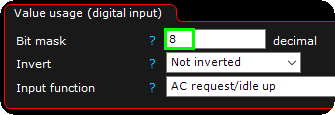When we apply the above example settings in MTune to trigger the AC request/idle up function in MaxxECU, from the fourth bit in the byte value.

mode

Momentary - if the matching bit is active, the controlled digital input function will be activated.

inverted Momentary - If the matching bit is active, the digital input will be deactivated, and the other way around if the queried bit is not active.

latching - If the matching bit is active, the controlled digital input will not be affected until a bit changes, then the digital input function will change state.

latching, keep state at power off - If the matching bit is active, the controlled digital input will not be affected until a bit changes, then the digital input function will change state. The bit state will be remembered at power off and used at power on.

input function

Use the digital input value directly on a MaxxECU input function. <-- use with caution...

## Old Examples (without the bit mask function)

Note: 0x430 is hexadecimal and it's the same as decimal value 1072.A display from a CAN analyzer on a Ford Mustang 2011, ID 0x430 (hexadecimal) is highlighted and in this example we pay attention

to the sixth byte (D6) which has default value of 0x10 (hexadecimal).Same CAN analyzer output on this Ford Mustang, but right now the Traction/EPS button is pressed. ID 0x430 (hexadecimal) and D6

value was now changed to 0x90 (hexadecimal).

Conclusion: When press and hold the Traction button, CAN ID 0x430, D6 byte is changed from 0x10 to 0x90.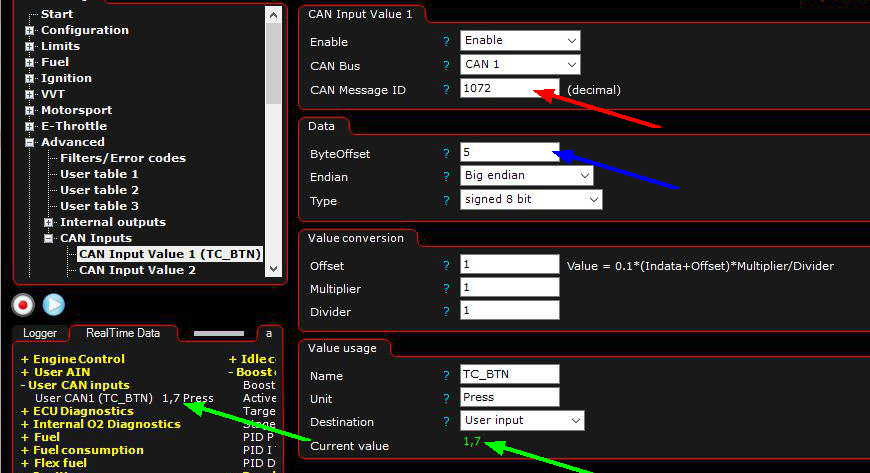Red arrow: Enter the decimal value which we will use as input 0x430(hex) = 1072(dec).

Blue arrow: Since the data we are looking for is in the sixth byte (D6) we will enter a byte ofset of 5 (skip the bytes before and just pay attention to the D6).

Green arrows: Indicated the converted value from vehicle CAN when Traction button is untouched, which can be used in MaxxECU for controlling functions

using internal output or virtual inputs.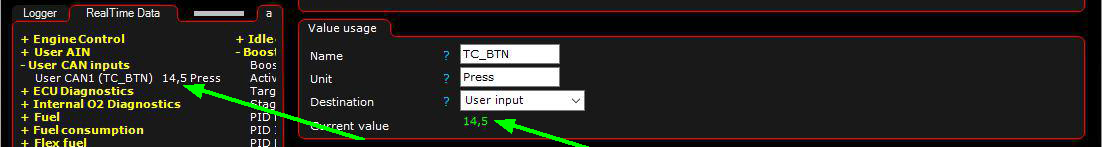Green arrows: Indicates the converted value from vehicle CAN when Traction button is pressed.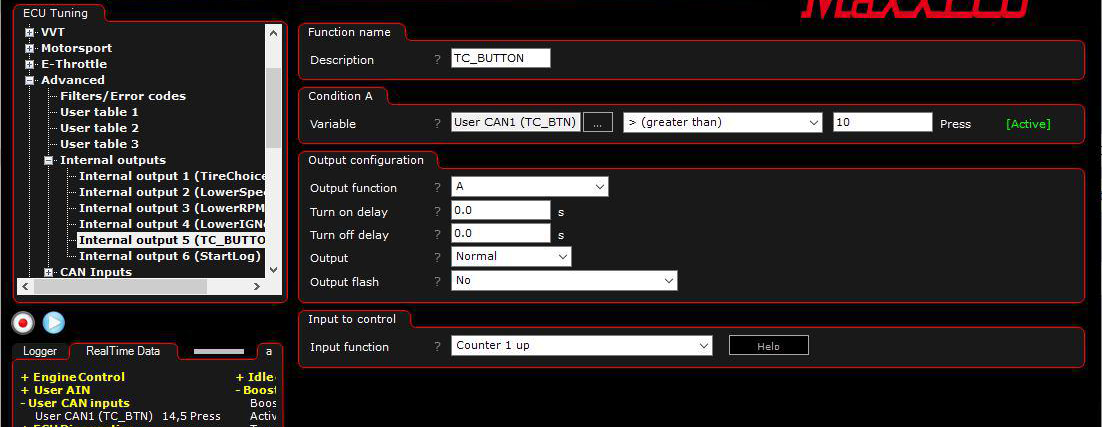In the above example the Ford Mustang Traction button only change value on the CAN when pressed, therefore we will use an internal output to take use of a

counter, which then later is triggering the Disable traction input function.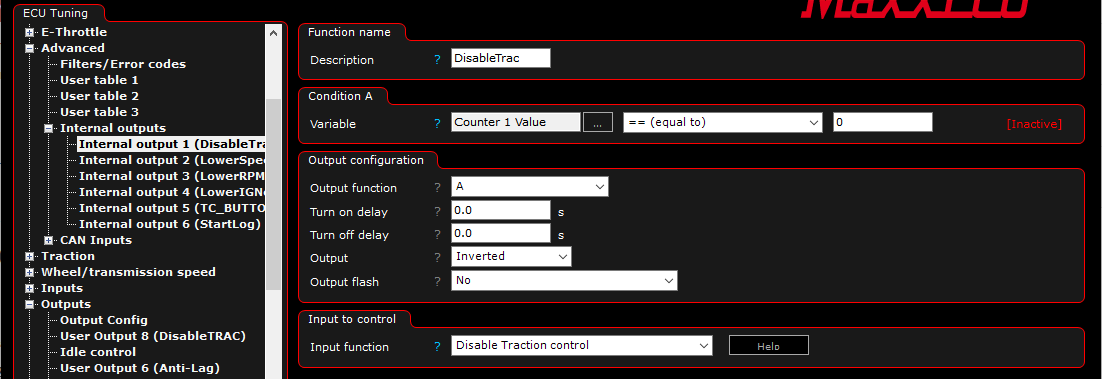Another Internal output is used to disable the Traction control feature (which is active all the time in the Traction control settings). Pay attention to the Inverted output since we

trigger the internal function Disable Traction control.

Note: This was just a simple example of what can be made using the User CAN input function, a lot of more advanced functions can be made.